• 2021-04-24 14:03:45

/*--------------------denghui-2015.11.16---------------------------*/

/*input： a int number n, means the number of node on the graph

n(row)*n(col) (int) , means the graph matrix

s(int), means the number of test data

s(row)*2(col) (int), means start and end

like this:

4

0 2 10 10000

2 0 7 3

10 7 0 6

1000 3 6 0

2

0 2

3 0

*/

#include

using namespace std;

#define MAX 30

void search(int Graph[][MAX], int Path[][MAX], int n);

void display(int Path[][MAX], int n);

void putout(int Path[][MAX], int start, int end);

int main()

{

int Graph[MAX][MAX];

int Path[MAX][MAX];

search(Graph, Path, n);

display(Path, n);

return 0;

}

{

int n;

cin >> n;

int i, j;

for (i = 0; i < n; ++i) {

for (j = 0; j < n; ++j) {

cin >> Graph[i][j];

}

}

return n;

}

void search(int Graph[][MAX], int Path[][MAX], int n)

{

int point[MAX][MAX];

int i, j, k;

for (i = 0; i < n; ++i) { // init

for (j = 0; j < n; ++j) {

point[i][j] = Graph[i][j];

Path[i][j] = -1;

}

}

for (i = 0; i < n; ++i) {

for (j = 0; j < n; ++j) {

for (k = 0; k < n; ++k) {

if (point[j][i] + point[i][k] < point[j][k]) {

point[j][k] = point[j][i] + point[i][k];

Path[j][k] = i;

}

}

}

}

}

void display(int Path[][MAX], int n)

{

int start, end, s;

cin >> s;

while (s > 0) {

s--;

cin >> start >> end;

putout(Path, start, end);

cout << end << endl;

}

}

void putout(int Path[][MAX], int start, int end)

{

if (Path[start][end] == -1) {

cout << start << endl;

} else {

putout(Path, start, Path[start][end]);

putout(Path, Path[start][end], end);

}

}

//end

/*=============================Denghui=================================*/

更多相关内容
• 求最短路径Floyd算法实现，无向图和有向图均适用。1先区别有向图和无向图，2输入顶点数和边数并检查合法性，3输入每边的起点、终点、权重并检查合法性，并初始化邻接矩阵和路径矩阵，4调用自定义函数Floyd
• Dijkstra和Floyd算法最短路径matlab实现，通信网作业
• #include <stdio.h> #include <...//二位数组保存每对顶点中的最短路径长度 int **nextVex;//二维数组保存vi到vj最短路径上vi后续节点的下标 }ShortPath; typedef struct GRAPHMATRIX_STRU {
#include <stdio.h>
#include <stdlib.h>
#define MAX 1000
typedef struct SHORTRSTPATH_STRU
{
int size;//图中节点的个数
int**pathLen;//二位数组保存每对顶点中的最短路径长度
int **nextVex;//二维数组保存vi到vj最短路径上vi后续节点的下标
}ShortPath;
typedef struct GRAPHMATRIX_STRU
{
int size;
int **graph;
}GraphMatrix;

GraphMatrix* InitGraph(int num)
{
int i,j;
GraphMatrix *graphMatrix=(GraphMatrix*)malloc(sizeof(GraphMatrix));
graphMatrix->size=num;
graphMatrix->graph=(int**)malloc(sizeof(int*)*graphMatrix->size);
for(i=0;i<graphMatrix->size;i++)
graphMatrix->graph[i]=(int*)malloc(sizeof(int)*graphMatrix->size);
for(i=0;i<graphMatrix->size;i++)
{
for(j=0;j<graphMatrix->size;j++)
graphMatrix->graph[i][j]=MAX;

}
return graphMatrix;
}
{
int vex1,vex2,weight;
scanf("%d%d%d",&vex1,&vex2,&weight);
while(vex1!=0||vex2!=0)
{
graphMatrix->graph[vex1][vex2]=weight;
scanf("%d%d%d",&vex1,&vex2,&weight);
}

}

ShortPath*floyd(GraphMatrix*graphMatrix)
{
int i,j,vex;
ShortPath *shortPath=NULL;
shortPath=(ShortPath*)malloc(sizeof(ShortPath));
shortPath->size=graphMatrix->size;
//分配空间二维数组
shortPath->nextVex=(int**)malloc(sizeof(int*)*shortPath->size);
for(i=0;i<shortPath->size;i++)
shortPath->nextVex[i]=(int*)malloc(sizeof(int)*shortPath->size);
//分配空间二维数组
shortPath->pathLen=(int**)malloc(sizeof(int*)*shortPath->size);
for(i=0;i<shortPath->size;i++)
shortPath->pathLen[i]=(int*)malloc(sizeof(int)*shortPath->size);
//初始化
for(i=0;i<shortPath->size;i++)
{
for(j=0;j<shortPath->size;j++)
{
//为了不破坏图的原有结构，使用辅助数组进行数据的迭代
shortPath->pathLen[i][j]=graphMatrix->graph[i][j];
if(shortPath->pathLen[i][j]==MAX)
shortPath->nextVex[i][j]=-1;
else
shortPath->nextVex[i][j]=j;
}
}
//正式开始计算
for(vex=0;vex<graphMatrix->size;vex++)
{
for(i=0;i<graphMatrix->size;i++)
{
for(j=0;j<graphMatrix->size;j++)
{
if(shortPath->pathLen[i][vex]==MAX||shortPath->pathLen[vex][j]==MAX)
continue;
//路径更短，则更新
if(shortPath->pathLen[i][vex]+shortPath->pathLen[vex][j]<shortPath->pathLen[i][j])
{
shortPath->pathLen[i][j]=shortPath->pathLen[i][vex]+shortPath->pathLen[vex][j];
shortPath->nextVex[i][j]=shortPath->nextVex[i][vex];
}
}
}

}
return shortPath;
}
int main()
{
//最短路径测试
GraphMatrix*graphMatrix=NULL;
ShortPath*shortPath=NULL;
int i,j,k,e,maxdistan,min=0;
graphMatrix=InitGraph(6);
shortPath=floyd(graphMatrix);//使用floyd算法
for(i=0;i<graphMatrix->size;i++)
{

for(j=0;j<graphMatrix->size;j++)
{
if(i!=j&&shortPath->pathLen[i][j]!=MAX)
{
printf("从%d到%d的最短路径长度为%d,具体路径为",i,j,shortPath->pathLen[i][j]);
printf("%d",i);//输出起点
k=i;//从起点出发，寻找完整路径
while(k!=j)
{
k=shortPath->nextVex[k][j];
printf("->%d",k);//将途径点输出
}
printf("\n");
/*if(min<shortPath->pathLen[i][j])
{
min=shortPath->pathLen[i][j];
maxdistan[i]=min;
}
if(i==0)
{
printf("%d->%d:%d",i,j,shortPath->pathLen[i][j]);
printf("\n");
printf("%d",i);
k=i;
while(k!=j)
{
k=shortPath->nextVex[k][j];
printf("->%d",k);
}
printf("\n");
}*/
}
}
}
return 0;
}展开全文数据结构
• Floyd算法又称为弗洛伊德算法、插点法，是一种利用动态规划的思想寻找给定的加权图中多源点之间最短路径的算法，与Dijkstra算法类似。该算法名称以创始人之一、1978年图灵奖获得者、斯坦福大学计算机科学系教授...

Floyd算法又称为弗洛伊德算法、插点法，是一种利用动态规划的思想寻找给定的加权图中多源点之间最短路径的算法，与Dijkstra算法类似。该算法名称以创始人之一、1978年图灵奖获得者、斯坦福大学计算机科学系教授罗伯特·弗洛伊德命名。

### 课题名称：设备更新，使总的支付费用最少

工厂的某台机器可连续工作四年，决策者每年年初都要决定机器是否需要更新。若更新，就要支付购置费用；若不更新，则要支付维修与运行费用，且随着机器使用年限的增加维修与运行费用逐年增多。计划期（4年）中每年年初的购置价格及各个年限内维修与运行费用由表1给出，

（1）试制订今后4年的机器更新计划，使总的支付费用最少。

表1

 第 i 年初 第1年初 第2年初 第3年初 第4年初 购置费（万元） 2.5 2.6 2.8 3.1 使用年限 1 2 3 4 使用年限下对应的每年的维修与运行费（万元） 1 1.5 2 4

可将此问题看成一个最短路问题。设 v1 和 v5 分别表示计划期的始点和终点（v5 可理解为第4年年末）。下图中各边的值(vi,vj)表示在第 i 年初购进的机器使用到第 j 年初（即第 j-1 年底），(vi,vj)的值可由表1的数据计算得到。因此，把求最优设备更新问题转化为求从 i 到 j 的最短路问题。

(vi,vj)的值计算示例：

（v1,v2） = 2.5（第1年初的购置费）+1（使用第一年的维修费） = 3.5；

（v3,v5） = 2.8（第3年初的购置费）+1（使用第一年的维修费）+1.5（使用第二年的维修费） = 5.3；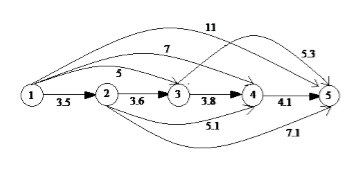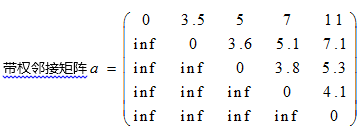Matlab求解

Matlab程序：

a=[0 3.5 5 7 11;inf 0 3.6 5.1 7.1;inf inf 0 3.8 5.3;inf inf inf 0 4.1;inf inf inf inf 0];
n=size(a,1);
D=a;
path=zeros(n,n);
for i=1:n
for j=1:n
if D(i,j)~=inf
path(i,j)=j;
end
end
end
for k=1:n
for i=1:n
for j=1:n
if D(i,k)+D(k,j)<D(i,j)
D(i,j)=D(i,k)+D(k,j);
path(i,j)=path(i,k);
end
end
end
end
D;path;


Matlab运行结果：

D =
0    3.5000    5.0000    7.0000   10.3000
Inf         0    3.6000    5.1000    7.1000
Inf       Inf         0    3.8000    5.3000
Inf       Inf       Inf         0    4.1000
Inf       Inf       Inf       Inf         0
>> path
path =
1     2     3     4     3
0     2     3     4     5
0     0     3     4     5
0     0     0     4     5
0     0     0     0     5


解得方案：最短路（v1,v3,v5），即计划期内机器更新最优计划为第1年、第3年初各购进一台新机器，4年总的支付费用为10.3万元。

## 新问题来了！

按照上述方案，第3年初购置新机器，那么旧机器是否可以低价处理掉呢？这样也更加符合实际。如果已知不同役龄机器年末的处理价格如表2所示，那么在这计划期内机器的最优更新计划又会怎样？

表2

 年度 第一年末 第二年末 第三年末 第四年末 机器处理价（万元） 2.0 1.6 1.3 1.1

类似上面的处理方式，可转化为求下图中从 v1 到 v5 的最短路问题。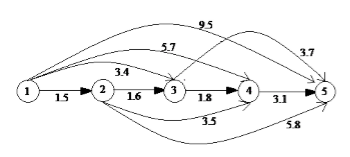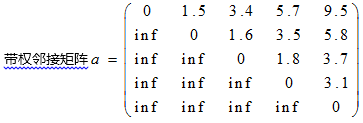Matlab求解

Matlab程序：

a=[0 1.5 3.4 5.7 9.5;inf 0 1.6 3.5 5.8;inf inf 0 1.8 3.7;inf inf inf 0 3.1;inf inf inf inf 0];
n=size(a,1);
D=a;
path=zeros(n,n);
for i=1:n
for j=1:n
if D(i,j)~=inf
path(i,j)=j;
end
end
end
for k=1:n
for i=1:n
for j=1:n
if D(i,k)+D(k,j)<D(i,j)
D(i,j)=D(i,k)+D(k,j);
path(i,j)=path(i,k);
end
end
end
end
D;path;


Matlab运行结果：

D =
0    1.5000    3.1000    4.9000    6.8000
Inf         0    1.6000    3.4000    5.3000
Inf       Inf         0    1.8000    3.7000
Inf       Inf       Inf         0    3.1000
Inf       Inf       Inf       Inf         0
>> path
path =
1     2     2     2     2
0     2     3     3     3
0     0     3     4     5
0     0     0     4     5
0     0     0     0     5


即最后解得：最短路 （v1,v2,v3,v5），即计划期内机器更新最优计划为第1年初购进一台新机器并在第2年初处理掉，第2年初购进一台新机器并在第3年初处理掉，第3年初购进一台新机器，4年总的支付费用为6.8万元。

内容很多，消化一下吧，希望可以为你所用！

展开全文数据建模
• 弗洛伊德算法是解决任意两点间的最短路径的一种算法，可以正确处理有向图或有向图或负权（但不可存在负权回路)的最短路径问题。该程序包括函数，主函数，以及打印出最短路径
• 基于matlab最短路径--floyd算法.doc 基于matlab最短路径-----Floyd算法在讲程序之前先看一个例子。例子：如图的交通网络，每条弧上的数字代表车辆在该路段行驶所需的时间。若有一批货物要从1号顶点运往11号顶点...基于matlab算最短路径--floyd算法.doc

基于matlab算最短路径-----Floyd算法在讲程序之前先看一个例子。例子：如图的交通网络，每条弧上的数字代表车辆在该路段行驶所需的时间。若有一批货物要从1号顶点运往11号顶点，问运货车应沿哪条线路行驶，才能最快地到达目的地？解答：我们可以根据上图建立一个矩阵，如行、列都表示的节点号，里面的对应的数字表示距离；inf的意思无穷大(不相邻的两个节点距离都假设为无穷大)，表示两个非直连节点间的距离。很明显，这是一个对称矩阵。1234567891011108infinfinfinf78infinfinf2803infinfinfinfinfinfinfinf3inf3056inf5infinfinfinf4infinf501infinfinfinfinf125infinf6102infinf7inf106infinfinfinf209inf3infinf77inf5infinf90infinfinfinf88infinfinfinfinfinf09infinf9infinfinfinf73inf902inf10infinfinfinfinfinfinfinf20211Infinfinf1210infinfinfinf20程序如下：第一步：先建立一个main.m的文件。10237411659813512210615887993227%*******************************************************clc;clear;globalarry;arry=zeros(0);%该数组是为了保存路径序号。start=( 请输入起点： );%起点(点的序号)ended=( 请输入终点： );%终点(点的序号)lines=load( Distance.txt );%导入数组。[D,path]=floyd(lines);%调用floyd程序算法。%读取D(start,ended)就可以得到最小距离。fprintf( \n最小距离是：%d\n ,D(start,ended));%下面是为了输出输出最短路径的程序。arry(1)=start;%路径第一个数字是开始点x=path(start,ended);%理解此处，必须要理解path数组。arry(2)=x;count=0;%以下程序，我是为了输出路径而写的，可以根据自己不同要求写。fori=3:11ifx~=endedx=path(x,ended);arry(i)=x;endcount=i;ifx==endedbreak;endendfprintf( \n最短路径是： );%fori=1:countfori=1:length(arry)ifarry(i)~=0;fprintf( %d ,arry(i));end%ifarry(i+1)~=0ifi~=length(arry)fprintf( - );endendfprintf( \n );%********************************************************第二步：先建立一个floyd.m的文件。%Floyd’sAlgorithmfunction[D,path]=fun(lines)a=lines;n=size(a,1);%设置D和path的初值D=a;path=zeros(n,n);fori=1:nforj=1:nifD(i,j)~=infpath(i,j)=j;%j是i的后继点endendend%做n次迭代，每次迭代均更新D(i,j)和path(i,j)fork=1:nfori=1:nforj=1:nifD(i,k)+D(k,j)

下载提示(请认真阅读)1.请仔细阅读文档，确保文档完整性，对于不预览、不比对内容而直接下载带来的问题本站不予受理。

2.下载的文档，不会出现我们的网址水印。

下载文档到电脑，查找使用更方便

8 积分

还剩页未读，继续阅读 关 键 词：基于MATLAB 最短路 Floyd算法 最短路径 MATLAB 基于Floyd算法 基于matlab floyd算法 matlab计算最短路径 Floyd 算法 最短路径算法 Matlab 最短路径Floyd算法matlab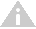蚂蚁文库所有资源均是用户自行上传分享，仅供网友学习交流，未经上传用户书面授权，请勿作他用。

关于本文

展开全文• 1)每对顶点的最短路径; 2)有向图、无向图和混合图; 算法思想:  直接在图的带权邻接矩阵中用插入顶点的方法依次递推地构造出n个矩阵D(1), D(2), …, D(n), D(n)是图的距离矩阵, 同时引入一个后继点矩阵记录两点...
• ## 【MATLAB】最短路径Floyd算法

千次阅读 热门讨论 2021-07-31 11:41:36
∙\bullet∙ 每队顶点的最短路径 ∙\bullet∙ 有向图、无向图和混合图 1.2算法思想 直接在图的带权邻接矩阵中用插入顶点的方法依次递推地构造出n个矩阵D(1)，D(2)…D(n)（每次加入一个点然后更新最短路径矩阵D），...
• 所有最短路径 在（一）中，我们获得了距离矩阵和路由矩阵（元胞） 这个一个无向图 这是上次算出的距离矩阵和路由矩阵，接下来介绍如何根据这个路由矩阵（元胞）写出所有最短路径 函数 function path=path_all(r, ...矩阵
• 本文将说明最短路径问题中的Floyd算法原理，以及如何用MATLAB实现。正文在这幅图中，如果我想知道任意两个点之间的最短距离，有什么好的办法吗？Floyd算法了解一下。在这个问题中，相邻两个点是“单向”的，也就是说...
• floyd算法解决最短路径问题，是一个函数文件，已标注了需要输入什么和会输出什么，使用时直接调用即可。
• 最短路径-任意两点间最短距离-Floyd算法matlab实现（详细教程） 目录 简介 核心思路 优缺点分析 算法过程 简介 Floyd算法又称为插点法，是一种利用动态规划的思想寻找给定的加权图中多源点之间最短路径的...动态规划
• 这里继续前面一篇《Python基于Floyd算法求解最短路径距离问题》的内容，这里要做的是Dijkstra算法，与Floyd算法类似，二者的用途均为求解最短路径距离，在图中有着广泛的应用，二者的原理都是老生常谈了，毕竟本科...
• 验证过正确的程序 kfunction [D,aver_D]=Aver_Path_Length) % 复杂网络中两节点的距离以及平均路径长度 % 求解算法首先利用Floyd算法求解出任意两节点的距离再距离的平均值得平均路径长度 % A网络图的邻接矩阵 %...
• Floyd算法又称为插点法，是解决任意两点间的最短路径的一种算法，可以正确处理无向图或有向图可以有负权重，但不可存在负权回路的最短路径问题。
• 先看看此篇博客，了解常规floyd算法是如何求最短路径的，搞懂D和R的意义，再往后看，否则看不懂 https://blog.csdn.net/kabuto_hui/article/details/82886826 要求所有最短路径，其实很简单。 不管有几条最短路径，...
• 图的最短路径算法 Dijkstra算法 Dijkstra算法研究的是从初始点到其他任一结点的最短路径，即单源最短路径问题，其对图的要求是不存在负权值的边。 Dijkstra算法主要特点是以起始点为中心向外层层扩展，直到扩展到...数据结构
• ## 最短路径-Floyd算法的matlab实现.md

万次阅读 多人点赞 2018-09-28 16:56:03
最短路径-Floyd算法matlab实现 ​ 弗洛伊德算法是解决任意两点间的最短路径的一种算法，可以正确处理有向图或有向图或负权（但不可存在负权回路)的最短路径问题。 ​ 在Floyd算法中一般有两个矩阵，一个距离矩阵D...
• 基于 matlabfloyd 算法 matlab 计算最短路径 function [d,path]=floyd(a,sp,ep) % floyd - 最短路问题 % % Syntax: [d,path]=floyd(a,sp,ep) % % Inputs: % a - 距离矩阵是指 i 到 j 之间的距离可以是有向的 % ...
• 使用了Floyd算法出了任意两点的距离矩阵和两点之间最短节点的矩阵，并用遗传算法创造四个父辈，对父辈遗传，且保持基因量相等，以最短空跑距离为适应度，筛选出最优秀的父辈子辈的其中有所有基因的四个。...
• 和Dijkstra算法一样，弗洛伊德（Floyd算法也是一种用于寻找给定的加权图中顶点间最短路径算法。该算法名称以创始人之一、1978年图灵奖获得者、斯坦福大学计算机科学系教授罗伯特·弗洛伊德命名。 弗洛伊德算法...
• 基于matlabfloyd算法 matlab计算最短路径.doc
• (小怒) 今天俺们一起学图论中的图和最短路径问题。 (深思) 图论中的图（Graph）是由若干给定的点及连接两点的线所构成的图形，这种图形通常用来描述某些事物之间的某种特定关系，用点代表事物，用连接两点的线表示...
• MATLAB实验报告,遗传算法最短路径以及函数最小值问题硕士生考查课程考试试卷考试科目： MATLAB教程考生姓名： 考生学号：学 院： 专 业：考 生 成 绩：任课老师 (签名)考试日期：20 年 月 日 午 时至 时《MATLAB...
• MATLAB中计算已知有向图、邻接矩阵情况下的最短路径...

# floyd算法求最短路径matlabmatlab 订阅